# Electronics and Communication Engineering - Exam Questions Papers

6.

For signal flow graph shown in following figure determine the ratio X5|X1. Use Mason's gain formula for signal flow graph.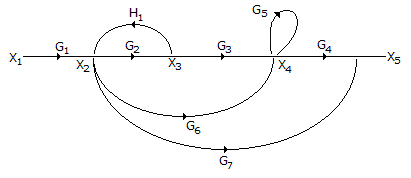A.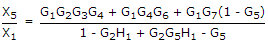B.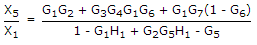C.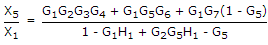D. None of these

Explanation:

Number of forward paths K = 3

T1 = G1G2G3G4

T2 = G1G6G4

T3 = G1G7

Number of loops

L1 = G2H1

L2= G5

Δ = 1 - [L1 + L2] + [L1 L2]

= 1 - [G2H1 + G5] + [G2G5H1]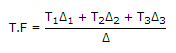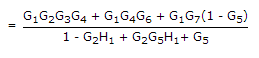.

7.

Consider following statements :

1. Maximum energy of electrons liberated photoelectrically is independent of light intensity
2. Maximum energy of electrons liberated photoelectrically varies nonlinearly with frequency of incident light.

 A. statement (1) is true and (2) is false B. statement (1) and (2) both are true C. statement (1) is false and (2) is true D. statement (1) and (2) both are false

Explanation:

Statement 2 is false because maximum energy of photons varies linearly with frequency of incident light. It can be shown as follows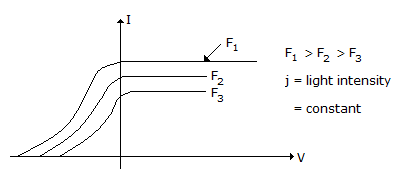Photocurrent Vs Anode voltage with frequency and incident light as parameter the light intensity is constant.

8.

The fourier transform of a voltage signal x(t) is X(f). The unit of X(f) is __________ .

 A. volts B. volt2 C. volt/sec D. volt-sec

Explanation:

F[x(t)] = X(f)

X(f) =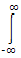x(t).e- jpft.dt

From above expression, the unit of x(t) x e-j2pft is volts and that of dt is seconds.

9.

A diode detector load consists of 0.01 μF capacitor in parallel with a 5 k ohms resistor. The maximum depth of modulation without diagonal clipping at modulating frequency of 1000 Hz and 10 kHz is

 A. 0.76, 0.24 B. 0.95, 0.3 C. 0.24, 0.76 D. 0.3, 0.95

Explanation: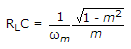At f = 1 KHz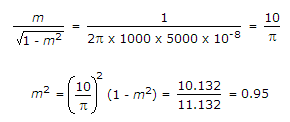Similarly for 10 kHz,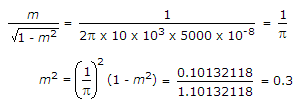10.

The inconsistency of Ampere's law can be removed by adding __________ to it.

 A. displacement current density alone. B. conduction current density alone. C. displacement and conduction current densities. D. none of these

Explanation:

The ampere's law is given as

∇.H = J

Taking divergence on both sides

∇.(∇xH) = ∇.J

i.e., ∇.(∇xH) = ∇.J = 0

divergence of curl is always zero

But ∇.J = -rv ... continuity equation.

Hence the inconsistency. It can be removed as follows

Let ∇ x H = J + G where G is some unknown quality.

Then ∇.(∇ x H) = ∇.(J x G) = 0

i.e. ∇.J + ∇.G = 0

i.e. ∇.J = -∇.G

or ∇.G = (-rv)

i.e. ∇.G = rv ...... Gauss's law in point form

Differentiating on both sides.

∇.D = rv

Comparing (i) and (ii), G = D

Thus ∇ x H = J + D

Where J is conduction current density and D is displacement current density which is added to remove the inconsistency of Ampere's law.

#### Current Affairs 2022

Interview Questions and Answers